# Multi-Class Hebrew Text Classification with Scikit-Learn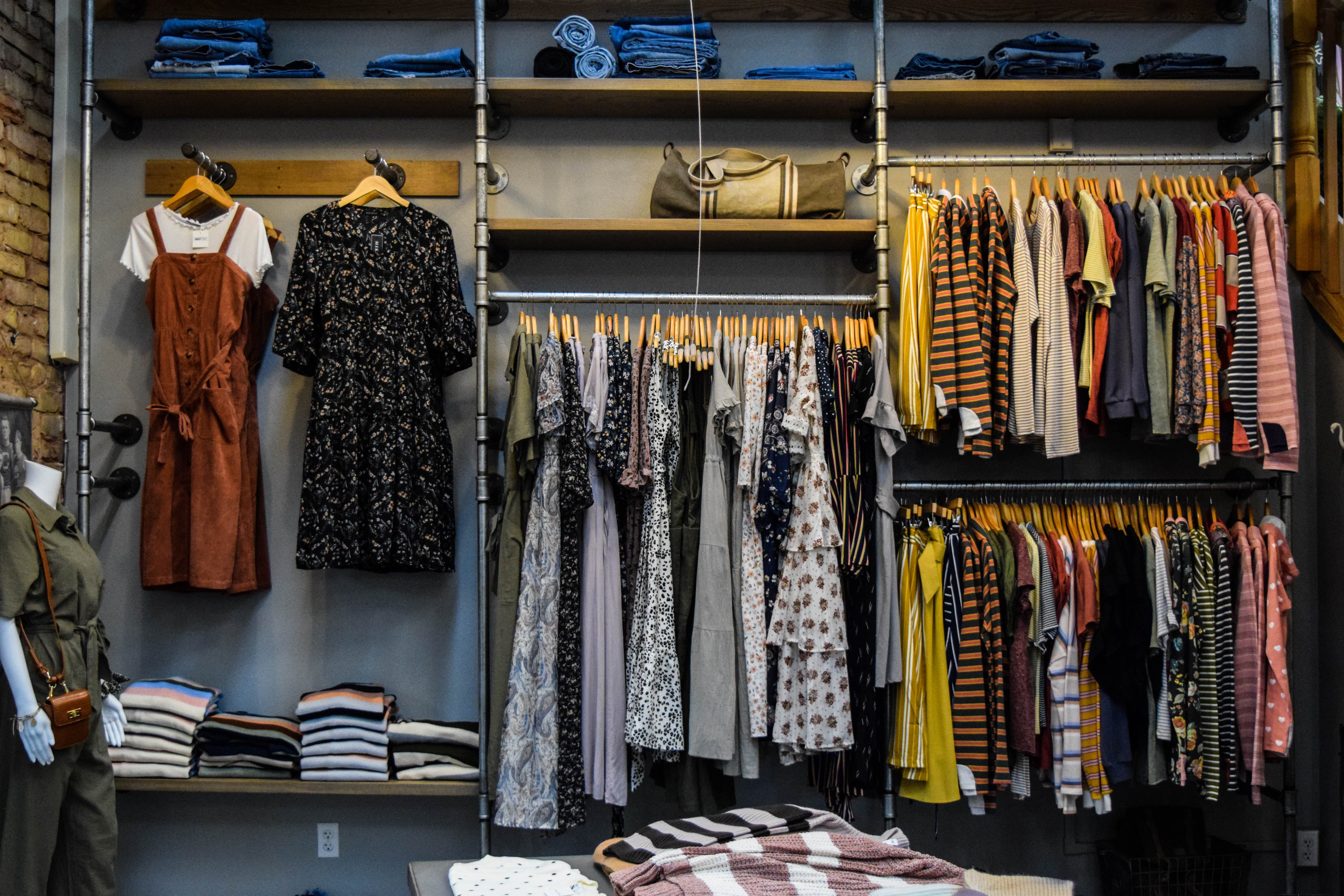Photo by Burgess Milner on Unsplash

In this article, we will classify women’s clothing product descriptions into 13 predefined classes. All descriptions are in Hebrew.

We will use two Scikit-Learn classifiers: Naive Bayes and Logistic Regression for multi-class machine learning algorithms.

# Goal

To predict the class of the product given its description.

# Data

The dataset is a collection of 24K women’s clothing product descriptions manually labeled. I have scrapped all data from popular Israel online fashion websites, and you can download it from here. We are going to use raw text directly rather than using a preprocessed text dataset.

## Load Data

Let’s take a snapshot of the our data:

`import pandas as pddf=pd.read_csv(“fashion_data.csv”,index_col=,header=)df=df.dropna()df=df.drop_duplicates()df=df.reset_index(drop=True)df.info()`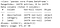Figure 1
`df.sample(10)`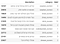Table 1

## Explore Data

Before we begin training machine learning models, we need to check the class distribution and the number of descriptions in each class:

`df_group = df.groupby("category")df_group = df_group.agg({"description": "nunique"})df_group = df_group.reset_index()df_group.head(13)`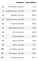Table 2

## Barplot

A barplot is one of the most common types of plot. It shows the relationship between a numerical variable and a categorical variable.

`import matplotlib.pyplot as pltimport matplotlib as mplmpl.rcParams['figure.dpi'] = 100df_group.plot(x='category', y='description', kind='bar', legend=False, grid=True, figsize=(8, 5))plt.title("Number of items per class")plt.ylabel('Number of items', fontsize=12)plt.xlabel('Class', fontsize=12)`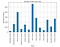Figure 1

## Word Cloud

A Wordcloud is a visual representation of text data. It displays a list of words, which represent the importance of each word by different sizes.

`from wordcloud import WordCloudimport matplotlib as mplmpl.rcParams[‘figure.dpi’] = 200def generate_word_cloud(text):   wordcloud = WordCloud(width = 600, height = 600,    background_color =’white’,    max_words=200,    min_font_size = 10,   font_path=’davidbd.ttf’).generate(text)      return wordcloudfrom bidi.algorithm import get_displaycleaned_text=list()for i,r in df.iterrows():   text=r[‘description’]   if isinstance(text, str):      bidi_text = get_display(text)      cleaned_text.append(bidi_text)wordcloud = generate_word_cloud(‘’.join(cleaned_text))plt.imshow(wordcloud, interpolation=’bilinear’)plt.axis(“off”)plt.show()`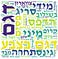Figure 2

# Classifier Models

Now we are ready for a machine learning algorithm.

## Split Data

First of all, we need randomly to split data into training and test sets.

We do it with Scikit-Learn train_test_split:

`from sklearn.model_selection import train_test_splitimport numpy as npX_train, X_test, y_train, y_test = train_test_split(np.array(df[‘description’]), np.array(df[‘category’]), test_size=0.33, random_state=42)X_train.shape, X_test.shape`

## Extract Features

To use our product descriptions in the model, we must encode each word as an integer value.

We do it with Scikit-Learn CountVectorizer:

`from sklearn.feature_extraction.text import CountVectorizercv=CountVectorizer(max_features=1500)cv_train_features = cv.fit_transform(X_train)cv_test_features = cv.transform(X_test)`

## Multinomial Naive Bayes

Multinomial Naive Bayes is a classification algorithm based on the Bayes’ theorem that derives the given feature vector’s probability of being associated with a class. This algorithm calculates each class’s probability for a given text and then output the class with the highest one.

We do it with Scikit-Learn MultinomialNB:

`from sklearn.naive_bayes import MultinomialNBmnb = MultinomialNB(alpha=1)mnb.fit(cv_train_features, y_train)mnb_test_score = mnb.score(cv_test_features, y_test)mnb_cv_scores = cross_val_score(mnb, cv_train_features, y_train, cv=5)mnb_cv_mean_score = np.mean(mnb_cv_scores)print('Cross Validation Accuracy (5-fold):', mnb_cv_scores)print('Mean of Cross Validation Accuracy:', mnb_cv_mean_score)print('Test Accuracy:', mnb_test_score)`Figure 3
`cv_pred_features = cv.transform([   ‘שמלה פרחונית ארוכה’   ,’מכנסי גינס’    ,’חולצה מכופתרת’   ])mnb.predict(cv_pred_features)`Figure 4

## Logistic Regression

Logistic regression is a linear classification algorithm that learns the probability of a sample belonging to a certain class. Logistic regression tries to find the optimal decision boundary that best separates the classes.

We do it with Scikit-Learn LogisticRegression:

`from sklearn.linear_model import LogisticRegressionlr = LogisticRegression(penalty='l2',solver='lbfgs', max_iter=100, C=1, random_state=42)lr.fit(cv_train_features, y_train)lr_test_score = lr.score(cv_test_features, y_test)lr_cv_scores = cross_val_score(lr, cv_train_features, y_train, cv=5)lr_cv_mean_score = np.mean(lr_cv_scores)print('Cross Validation Accuracy (5-fold):', lr_cv_scores)print('Mean of Cross Validation Accuracy:', lr_cv_mean_score)print('Test Accuracy:', lr_test_score)`Figure 5
`cv_pred_features = cv.transformcv.transform([   ‘שמלה פרחונית ארוכה’   ,’מכנסי גינס’    ,’חולצה מכופתרת’   ])lr.predict(cv_pred_features)`Figure 6

You can found the source code on Github. I look forward to hearing any feedback and questions.

Data Scientist